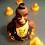### Overview

In a previous article, I outlined why BigDecimal is not the answer most of the time.  While it is possible to construct situations where double produces an error, it is also just as easy to construct situations were BigDecimal get an error.

### BigDecimal is easier to get right, but easier to get wrong.

The anecdotal evidence is that junior developers don't have as much trouble getting BigDecimal right as they do getting double with rounding right.  However, I am sceptical of this because in BigDecimal it is much easier for an error to go unnoticed as well.

Lets take this example where double produces an incorrect answer.

double d = 1.00;
d /= 49;
d *= 49 * 2;
System.out.println("d=" + d);

BigDecimal bd = BigDecimal.ONE;
bd = bd .divide(BigDecimal.valueOf(49), 2, BigDecimal.ROUND_HALF_UP);
bd = bd.multiply(BigDecimal.valueOf(49*2));
System.out.println("bd=" + bd);

prints

d=1.9999999999999998
bd=1.96

In this case, double looks wrong, it needs rounding which would give the correct answer of 2.0. However the BigDecimal looks right, but it isn't due to representation error.  We could change the division to use more precision, but you will always get a representation error, though you can control how small that error is.

### You have to ensure numbers are real and use rounding.

Even with BigDecimal, you have to use appropriate rounding.  Lets say you have a loan for \$1,000,000 and you apply 0.0005% interest per day.  The account can only have a whole number of cents, so rounding is needed to make this a real amount of money.  If don't do this how long does it take to make a 1 cent difference?

double interest = 0.0005;
BigDecimal interestBD = BigDecimal.valueOf(interest);

double amount = 1e6;
BigDecimal amountBD = BigDecimal.valueOf(amount);
BigDecimal amountBD2 = BigDecimal.valueOf(amount);

long i = 0;
do {
System.out.printf("%,d: BigDecimal: \$%s, BigDecimal: \$%s%n", i, amountBD, amountBD2);
i++;
.setScale(2, BigDecimal.ROUND_HALF_UP));

} while (amountBD2.subtract(amountBD).abs()
.compareTo(BigDecimal.valueOf(0.01)) < 0);
System.out.printf("After %,d iterations the error was 1 cent and you owe %s%n", i, amountBD);

prints finally.

8: BigDecimal: \$1004007.00,
BigDecimal: \$1004007.00700437675043756250390625000000000000000
After 9 iterations the error was 1 cent and you owe 1004509.00

You could round the result but this hide the fact you are off by a cent even though you used BigDecimal.

### double eventually has a representation error

Even if you use appropriate rounding, double will give you an incorrect result.  It is much later than the previous example.

double interest = 0.0005;
BigDecimal interestBD = BigDecimal.valueOf(interest);
double amount = 1e6;
BigDecimal amountBD = BigDecimal.valueOf(amount);
long i = 0;
do {
System.out.printf("%,d: double: \$%.2f, BigDecimal: \$%s%n", i, amount, amountBD);
i++;
amount = round2(amount + amount * interest);

.setScale(2, BigDecimal.ROUND_HALF_UP));
} while (BigDecimal.valueOf(amount).subtract(amountBD).abs()

.compareTo(BigDecimal.valueOf(0.01)) < 0);
System.out.printf("After %,d iterations the error was 1 cent and you owe %s%n", i, amountBD);

prints finally

22,473: double: \$75636308370.01, BigDecimal: \$75636308370.01
After 22,474 iterations the error was 1 cent and you owe 75674126524.20

From an IT perspective we have an error of one cent, from a business perspective we have a client who has made no repayments for more than 9 years and owes the bank \$75.6 billion, enough to bring down the bank.  If only the IT guy had used BigDecimal !?

### Conclusion

My final recommendation is that you should use what you feel comfortable with, don't forget about rounding, do use real numbers, not whatever the mathematics produces e.g. can I have fractions of a cent, or can I trade fractions of share.  Don't forget about the business perspective.  You might find that BigDecimal makes more sense for your company, your project or your team.

Don't assume BigDecimal is the only way, don't assume the problems double faces don't apply also to BigDecimal. BigDecimal is not a ticket to best practice coding, because complacency is a sure way of introducing errors.

1.I probably missed the whole point, but if we remove the
.setScale(2, BigDecimal.ROUND_HALF_UP)
in the last snippet we will also get 9 iterations only. In the former we compared BigDecimal with rounded BigDecimal and in the latter we compare rounded double with rounded BigDecimal. (I assume the round2() is equivalent of round6() from your previous post with 1e2 factor.)

2.This comment has been removed by the author.

3.Neither can handle irrational numbers. But BigDecimal handles adding 0.1 without rounding.

double d = 0;
BigDecimal bd = BigDecimal.ZERO;

for (int i=0; i<3; i++)
{
d += .1;
System.out.println("bd=" + bd);
System.out.println("d=" + d);
}

4.Neither can handle rational numbers, e.g., 1/3.

And I don't buy your example: With double I need rounding, but the code is still shorter, faster, and more readable than when using BigDecimal.

5.To me it looks like the constraints of the system here are the problem, not BigDecimal. I know this is an example to show how both can cause issues, but precision is highly unlikely to be so constrained in the real world.

6.This comment has been removed by a blog administrator.